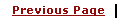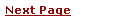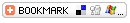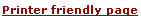C - Storage ClassesLearning C
C Function References
C Useful Resources# C - Storage ClassesA storage class defines the scope (visibility) and life time of variables and/or functions within a C Program.

There are following storage classes which can be used in a C Program

• auto

• register

• static

• extern

## auto - Storage Class

auto is the default storage class for all local variables.

 ``` { int Count; auto int Month; } ```

The example above defines two variables with the same storage class. auto can only be used within functions, i.e. local variables.

## register - Storage Class

register is used to define local variables that should be stored in a register instead of RAM. This means that the variable has a maximum size equal to the register size (usually one word) and cant have the unary '&' operator applied to it (as it does not have a memory location).

 ``` { register int Miles; } ```

Register should only be used for variables that require quick access - such as counters. It should also be noted that defining 'register' goes not mean that the variable will be stored in a register. It means that it MIGHT be stored in a register - depending on hardware and implimentation restrictions.

## static - Storage Class

static is the default storage class for global variables. The two variables below (count and road) both have a static storage class.

 ``` static int Count; int Road; { printf("%d\n", Road); } ```

static variables can be 'seen' within all functions in this source file. At link time, the static variables defined here will not be seen by the object modules that are brought in.

static can also be defined within a function. If this is done the variable is initalised at run time but is not reinitalized when the function is called. This inside a function static variable retains its value during vairous calls.

 ``` void func(void); static count=10; /* Global variable - static is the default */ main() { while (count--) { func(); } } void func( void ) { static i = 5; i++; printf("i is %d and count is %d\n", i, count); } This will produce following result i is 6 and count is 9 i is 7 and count is 8 i is 8 and count is 7 i is 9 and count is 6 i is 10 and count is 5 i is 11 and count is 4 i is 12 and count is 3 i is 13 and count is 2 i is 14 and count is 1 i is 15 and count is 0 ```

NOTE : Here keyword void means function does not return anything and it does not take any parameter. You can memoriese void as nothing. static variables are initialized to 0 automatically.

Definition vs Declaration : Before proceeding, let us understand the difference between defintion and declaration of a variable or function. Definition means where a variable or function is defined in realityand actual memory is allocated for variable or function. Declaration means just giving a reference of a variable and function. Through declaration we assure to the complier that this variable or function has been defined somewhere else in the program and will be provided at the time of linking. In the above examples char *func(void) has been put at the top which is a declaration of this function where as this function has been defined below to main() function.

There is one more very important use for 'static'. Consider this bit of code.

 ``` char *func(void); main() { char *Text1; Text1 = func(); } char *func(void) { char Text2="martin"; return(Text2); } ```

Now, 'func' returns a pointer to the memory location where 'text2' starts BUT text2 has a storage class of 'auto' and will disappear when we exit the function and could be overwritten but something else. The answer is to specify

 ``` static char Text="martin"; ```

The storage assigned to 'text2' will remain reserved for the duration if the program.

## extern - Storage Class

extern is used to give a reference of a global variable that is visible to ALL the program files. When you use 'extern' the variable cannot be initalized as all it does is point the variable name at a storage location that has been previously defined.

When you have multiple files and you define a global variable or function which will be used in other files also, then extern will be used in another file to give reference of defined variable or function. Just for understanding extern is used to decalre a global variable or function in another files.

File 1: main.c

 ``` int count=5; main() { write_extern(); } ```

File 2: write.c

 ``` void write_extern(void); extern int count; void write_extern(void) { printf("count is %i\n", count); } ```

Here extern keyword is being used to declare count in another file.

Now compile these two files as follows

 ``` gcc main.c write.c -o write ```

This fill produce write program which can be executed to produce result.

Count in 'main.c' will have a value of 5. If main.c changes the value of count - write.c will see the new value